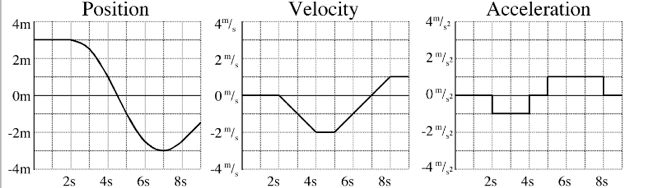One-Dimensional Motion Questions

Average Speed:

1.  Estimate the average speed of the object in Graph A, B, and C.  Explain your reasoning.

Change in Velocity:

2.  Rank the four situations from high to low AND calculate the change in velocity in each situation.

Average Acceleration:

3.  Rank from highest to lowest for average acceleration AND calculate the acceleration on each graph.

4.  Which graph(s) show(s) constant velocity?  variable velocity?

5.  Which graph(s) show(s) negative velocity? positive velocity? zero velocity?

6.  Which graph(s) show(s) negative displacement? positive displacement? zero displacement?

7.  CALCULATE the displacement for each graph.

8. For the three graphs below, EXPLAIN what each segment (four of them) means.  Look carefully at the vertical labels. There are corresponding time frames for each graph. Use terms such as constant, variable, negative, positive, and/or zero to describe the displacement, velocity and acceleration in each time frame.(source)